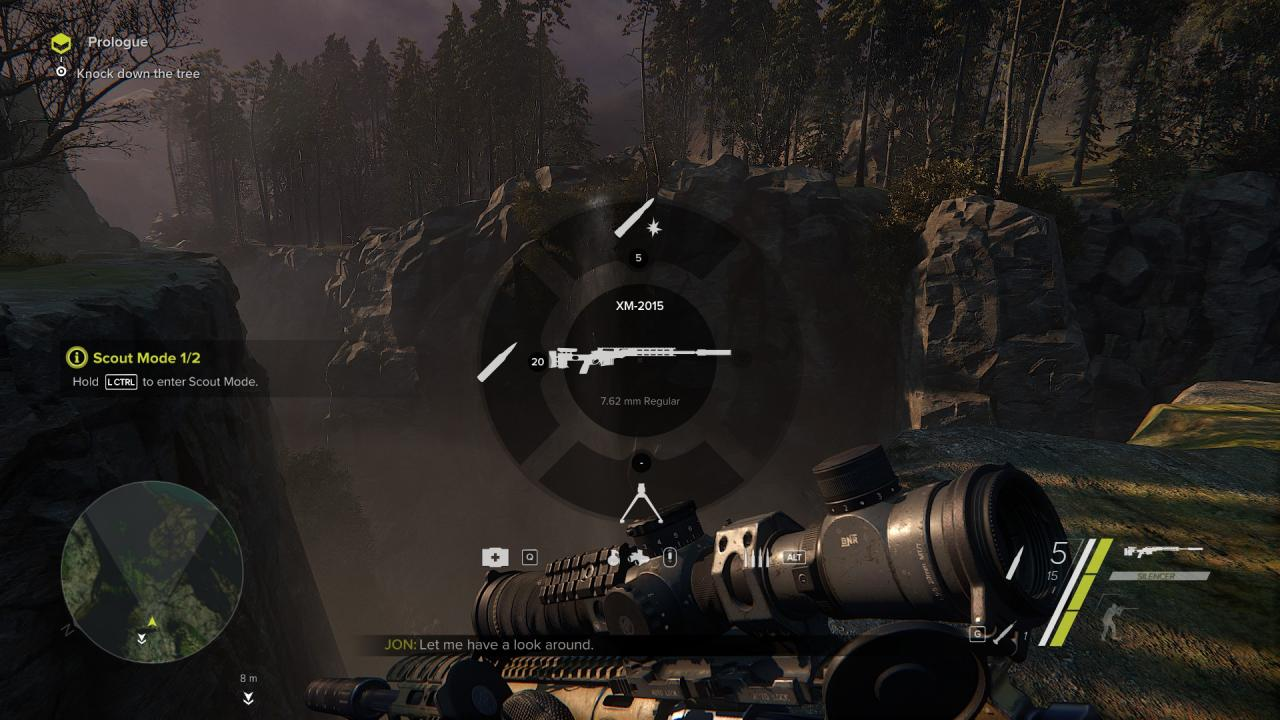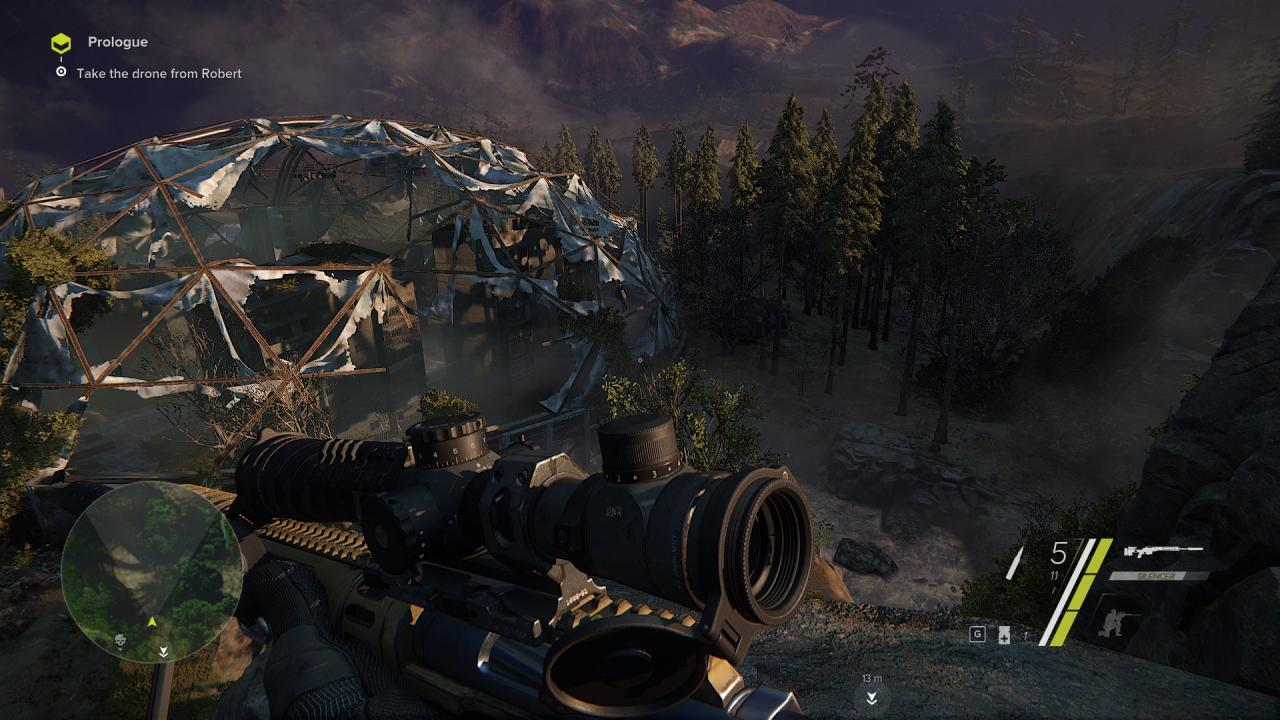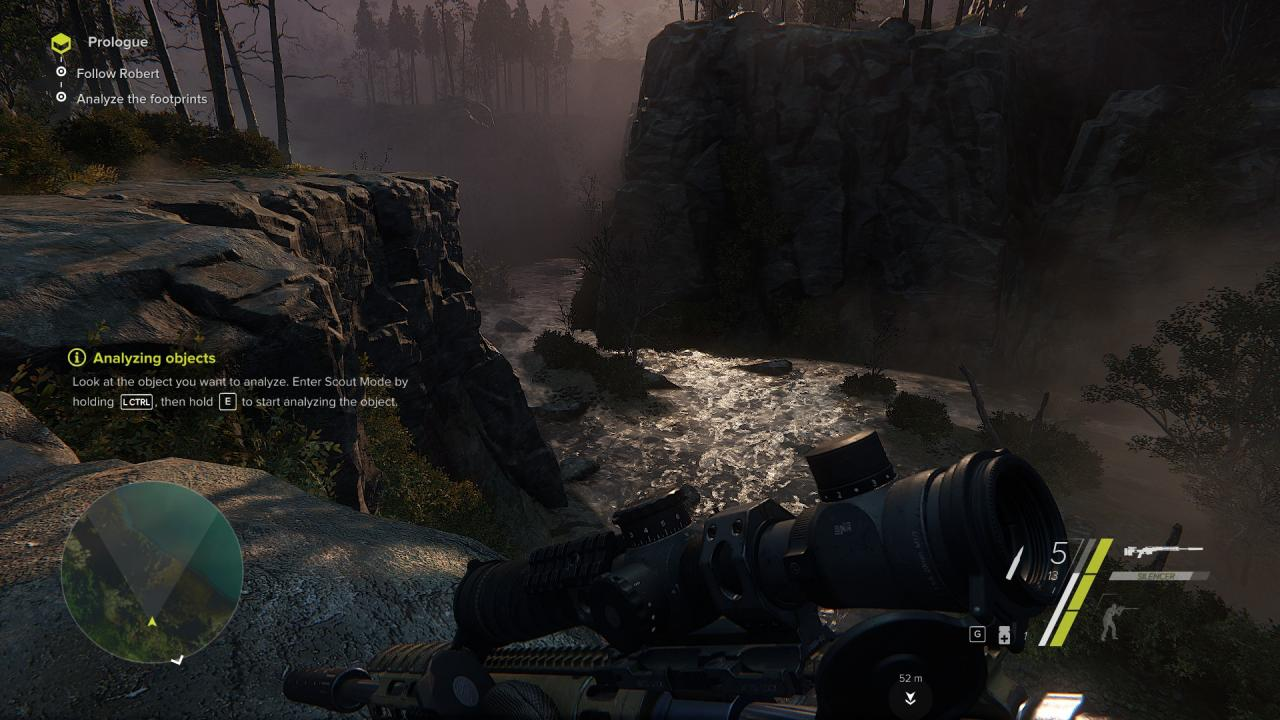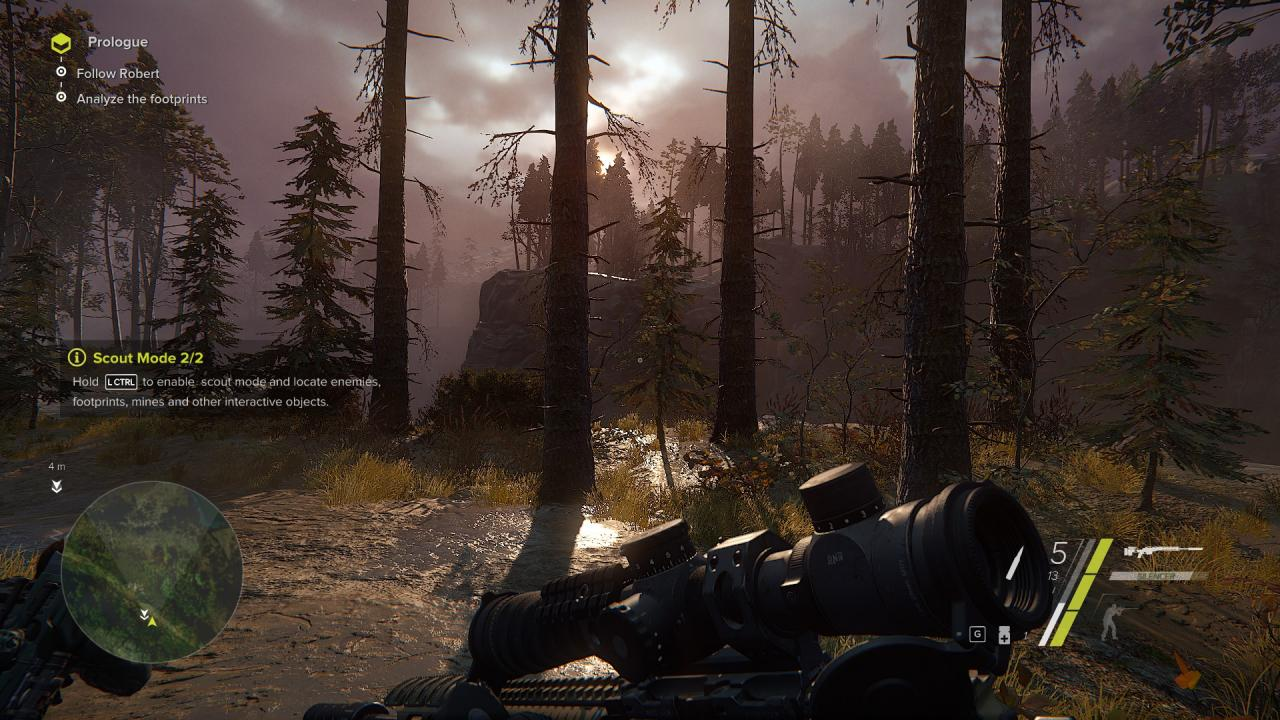# Sniper Ghost Warrior 3: Graphical Fidelity Settings

This guide will alter settings in your autoexec.cfg file in order to achieve better graphical fidelity in the game.

These settings will require appropriate PC hardware in order to achieve the intended results. Therefore, it is recommended that you system requirements meet the Recommended System Requirements for the game.

Autoexec.cfg Contents
Copy and paste the following into an autoexec.cfg in the game main folder.

Once copy and pasted, save the file, and change its properties to “read only”.

;Graphics
sys_spec = 4
sys_spec_ObjectDetail = 4
sys_spec_Full = 4
sys_spec_GameEffects = 4
sys_spec_MotionBlur = 4
sys_spec_ObjectDetail = 4
sys_spec_Particles = 4
sys_spec_Physics = 4
sys_spec_PostProcessing = 4
sys_spec_Shading = 4
sys_spec_Shadows = 4
sys_spec_Sound = 4
sys_spec_Texture = 4
sys_spec_TextureResolution = 4
sys_spec_VolumetricEffects = 4
sys_spec_Quality = 4
sys_spec_Water = 4
sys_spec_Light = 4
sys_spec_RayTracing = 4

;Clouds
e_Clouds = 1
r_CloudsUpdateAlways = 1
e_ShadowsClouds = 1
e_SkyType = 1
e_SkyUpdateRate = 100
e_SkyQuality = 1
e_SkyBox = 1

;Motion Blur
cl_motionBlur = 2
ca_MotionBlurMovementThreshold = 0.001
r_MotionBlur = 2
r_MotionBlurGBufferVelocity = 1
r_MotionBlurQuality = 2
r_MotionBlurShutterSpeed = 13
r_MotionBlurThreshold = 0.0001
r_MotionBlurMaxViewDist = 32
r_MotionBlurGBufferVelocity = 1

;Color
r_ColorGradingCharts = 0
r_CloakHeatScale = 0.25
r_CloakLightScale = 0.25
r_CloakRefractionFadeByDist = false
r_CloakRefractionFadeMaxDistSq = 25.0
r_CloakRefractionFadeMinDistSq = 1.0
r_CloakRefractionFadeMinValue = 0.2
r_CloakTransitionLightScale = 1.0
r_ColorBits = 32
r_ColorGrading = 2
r_ColorGradingDof = 1
r_ColorGradingFilters = 1
r_ColorGradingLevels = 1
r_ColorGradingSelectiveColor = 1
r_DetailDistance = 20
r_DetailNumLayers = 2
r_DepthBits = 32
r_CustomVisions = 1

;Shadows
e_shadows = 1
e_ShadowsMasksLimit = 0
e_ShadowsCastViewDistRatio = 20
e_ShadowsConstBiasHQ = 1
e_shadows_clouds = 1
e_shadows_debug = 0
e_shadows_from_terrain_in_all_lods = 1
e_shadows_frustums = 0
e_shadows_max_texture_size = 4096
e_ShadowsMaxTexRes = 4096
e_ShadowsOcclusionCulling = 1
e_shadows_occ_check = 0
e_shadows_occ_cutCaster = 0
e_shadows_on_alpha_blended = 1
e_shadows_slope_bias = -4
e_shadows_water = 1
e_ShadowsOnWater = 1
e_ShadowsMasksLimit = 2
e_volobj_shadow_strength = 0.4
r_ShadowsBias = 1
r_ShadowsDeferredMode = 1
r_ShadowsDepthBoundN = 1
r_ShadowsForwardPass = 0
r_ShadowsGridAligned = 0
r_ShadowsMaskDownScale = 0
r_ShadowsMaskResolution = 8192
r_ShadowsNearestMapResolution = 8192
r_ShadowsParticleNormalEffect = 1
r_ShadowsSlopeScaleBias = 1.8
r_ShadowsStencilPrePass = 1
r_ShadowBlur = 3
r_ShadowBluriness = 1
r_ShadowGen = 1
r_ShadowGenGS = 1
r_ShadowGenMode = 1
r_ShadowJittering = 1
r_ShadowPass = 1
r_ShadowsParticleNormalEffect = 1
r_ShadowsStaticMapResolution = 8192
r_ShadowsPCFiltering = 1
r_ShadowTexFormat = 1
r_VarianceShadowMapBlurAmount = 1
r_DrawNearShadows = 1
r_UseShadowsPool = 1
r_ShadowsPCFiltering = 1

;Fog
e_Fog = 1
r_FogShadows = 2
r_FogShadowsWater = 2

;Foliage, Vegetation and Terrain
e_foliage_wind_activation_dist
e_vegetation_use_terrain_color = 1
e_view_dist_ratio_detail = 45
e_materials = 1
e_on_demand_maxsize
e_phys_foliage = 2
e_proc_vegetation
e_detail_materials = 1
e_detail_materials_view_dist_xy = 50
e_detail_materials_view_dist_z = 100
e_dissolve = 0
e_ObjQuality = 4
e_TerrainOcclusionCullingVersion = 1
e_PhysFoliage = 2
r_TerrainAO = 7
r_TerrainAO_FadeDist = 0
r_TessellationTriangleSize = 10
r_DetailDistance = 8

;Lights and Global Illumination
e_DynamicLights = 1
e_GI = 1
e_GIAmount = 0.6
e_GIMaxDistance = 100
e_GIIterations = 32
e_GINumCascades = 1
e_LightVolumes = 1
e_GIGlossyReflections = 1
e_GIMaxDistance = 150
e_GIRSMSize = 512
e_GISecondaryOcclusion = 1
e_Gsm_Cache = 1
e_gsm_cache_lod_offset = 3
e_gsm_combined = 0
e_gsm_depth_bounds_debug = 0
e_gsm_lods_num = 5
e_gsm_range = 5
e_gsm_range_step = 5
e_gsm_scatter_lod_dist = 100
e_gsm_view_space = 0
e_LightVolumes = 1
r_Beams = 1
r_BeamsDistFactor = 0.01
r_BeamsHelpers = 1
r_BeamsMaxSlices = 300
r_BeamsSoftClip = 0
r_Glow = 1
r_FlareHqShafts = 1
r_Flares = 1
r_FlaresChromaShift = 6
r_FlaresTessellationRatio = 1
r_LightPropagationVolumes = 1
r_DeferredShadingLights = 1
r_DeferredShadingSortLights = 1
r_DeferredShadingSSS

;Depth of Field
r_DepthOfField = 2
r_DepthOfFieldDilation = 0
r_DepthOfFieldBokeh = 1
r_DepthOfFieldBokehQuality = 1
r_DrawNearFoV = 60

;HDR
r_HDRBloomRatio = 1
r_HDRBrightLevel = 1.25
r_HDRGrainAmount = 0
r_HDRRendering = 2
r_HDRTexFormat = 1
r_HDRVignetting = 1
r_HDRRangeAdapt = 1
r_HDRRangeAdaptLBufferMax = 0.25
r_HDRRangeAdaptLBufferMaxRange = 2.0
r_HDRRangeAdaptMax = 1.0
r_HDRRangeAdaptMaxRange = 4.0
r_HDREyeAdaptationCache = 0
r_HDREyeAdaptationFactor = 0.9
r_HDREyeAdaptationSceneKeyMode = 1
r_HDREyeAdaptationMode = 1
r_HDREyeAdaptationSpeed = 3

;Anti Aliasing
r_MSAA = 1
r_MSAA_quality = 8
r_MSAA_samples = 8
r_AntialiasingMode = 3
r_AntialiasingTAAMode = 3
;r_AntialiasingTSAAMipBias = -3
;r_AntialiasingTSAASubpixelDetection = 1
;r_AntialiasingTSAASmoothness = 0.15
r_AntialiasingTAASharpening = 1
r_AntialiasingModeSCull = 1
r_AntialiasingTAAFalloffHiFreq = 0.5
r_AntialiasingTAAFalloffLowFreq = 0.5
r_UseEdgeAA = 2

;Shaders
r_DeferredShadingAmbient = 1
r_DeferredShadingAmbientLights = 1
r_DeferredShadingAreaLights = 1
r_DeferredShadingEnvProbes = 1
r_DeferredShadingLights = 1
r_DeferredShadingLightVolumes = 1
r_DeferredShadingSortLights = 1
r_DeferredShadingSSS = 1
r_DeferredShadingTiledHairQuality = 2
r_ShadersAsyncActivation = 1
r_ShadersDX11 = 1
r_OptimiseShaders = 1
r_ParticlesRefraction = 1
r_ParticlesTessellation = 1
r_ParticlesSoftIsec = 1
r_PostProcessEffects = 1
r_PostProcessFilters = 1
r_PostProcessGameFx = 1
r_ProfileShadersSmooth = 10
r_PrecacheShaderList
r_PrecacheShaders
r_Reflections = 1
r_ReflectionsQuality = 3
r_RefractionPartialResolves = 1
q_ShaderFX = 3
q_Renderer = 3
q_ShaderGeneral = 3
q_ShaderGlass = 3
q_ShaderIce = 3
q_ShaderMetal = 3
q_ShaderPostProcess = 3
q_ShaderSky = 3
q_ShaderShadow = 3
q_ShaderTerrain = 3
q_ShaderVegetation = 3
q_ShaderWater = 3
q_ShaderHDR = 3

;Particles
e_gsm_range_step_terrain = 16
e_gsm_scatter_lod_dist = 100
e_gsm_stats = 0
e_gsm_view_space = 0
e_ParticlesMaxScreenFill = 160
e_particles_preload = 1
e_ParticlesQuality = 4
e_ParticlesThread = 1
e_ParticlesGI = 1
e_ParticlesMotionBlur = 1
e_ParticlesObjectCollisions = 2
e_ParticlesShadows = 1
r_ParticlesTessellation = 1
r_ParticlesTessellationTriSize = 1
r_ParticlesRefraction = 1
r_ParticlesAmountGI
r_ParticlesSoftIsec = 1
e_ParticlesMinDrawPixels = 1

;PostProcessing
r_PostProcessEffectsFilters = 1
r_PostProcessEffects = 1
r_PostProcessFilters = 1
r_PostProcessEffectsGameFx = 1
r_PostProcessHUD3D = 1
r_PostProcessHUD3DCache = 1
r_PostProcessHUD3DGlowAmount = 1.0
r_PostProcessHUD3DShadowAmount = 1.7
r_PostProcessEffectsParamsBlending = 1
r_PostprocessParamsBlendingTimeScale = 12
r_PostProcessNanoGlassDebugView = 0
r_PostProcessParamsBlending = 1

;Physics
sys_spec_Physics = 4
ca_UsePhysics = 1
es_OnDemandPhysics = 1
e_particles_lights = 1
e_OnDemandPhysics = 1
e_ObjectLayersActivationPhysics = 1
gpu_particle_physics = 1

;Textures
r_TexAnisotropy = 16
r_TexMaxAnisotropy = 16
r_TexMinAnisotropy = 15
r_TexSkyResolution = 2
r_TextureCompressor = 0
r_TexturesFilteringQuality = 0
r_TexturesStreamPoolSize = 4096
r_TexturesStreaming = 0
r_TexturesStreamingMipBias = -4
r_TexturesStreamingMipFading = 1
r_TexturesStreamingMaxRequestedJobs = 32
r_TexturesStreamingMaxRequestedMB = 4.0
r_TextureLodDistanceRatio = 0
r_EnvTexResolution = 3
r_EnvCMResolution = 2
r_DetailTextures = 1
r_ShowDynTextures = 1
r_EnvTexUpdateInterval = 0.5
e_MaxViewDistance = 1
e_MaxViewDistSpecLerp = 1

;Decals
e_decals = 1
e_render = 1
e_DecalsAllowGameDecals = 1
e_DecalsDefferedDynamic = 1
e_decals_hit_cache
e_decals_life_time_scale
e_decals_merge
e_decals_neighbor_max_life_time = 0
e_decals_overlapping
e_decals_precreate
e_PreloadDecals = 1
e_decals_range
e_detail_materials_view_dist_z

;Particles
e_particles_lod = 0
e_particles_max_emitter_draw_screen = 4
e_particles_min_draw_pixels = 1
e_particles_object_collisions = 1
e_particles_preload = 1
e_particles_quality = 4
e_ParticlesThread = 1
e_ParticlesAnimBlend = 1
e_ParticlesGI = 1
e_ParticlesLights = 1
e_ParticlesMotionBlur = 1
e_ParticlesSo

Screenshots